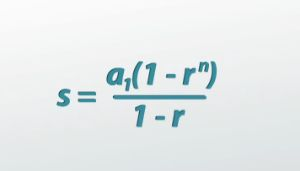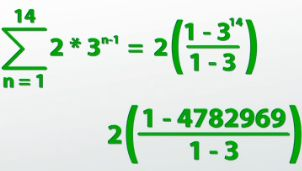# How to Calculate a Geometric Series

An error occurred trying to load this video.

Try refreshing the page, or contact customer support.

Coming up next: Arithmetic and Geometric Series: Practice Problems

### You're on a roll. Keep up the good work!

Replay
Your next lesson will play in 10 seconds
• 0:56 Proving the Formula
• 4:03 Solving the Formula
• 5:16 Proving Infinite Series
• 7:00 Solving Infinite Series
• 8:38 Lesson Summary

Want to watch this again later?

Timeline
Autoplay
Autoplay
Speed

#### Recommended Lessons and Courses for You

Lesson Transcript
Instructor: Jeff Calareso

Jeff teaches high school English, math and other subjects. He has a master's degree in writing and literature.

If you're not satisfied with knowing how many views your YouTube video will get any one day and need to know how many total views you're getting, geometric series are for you! Learn more in this lesson.

## How Many Views Will We Get?

In a previous lesson about geometric sequences, we saw that if a YouTube video gets two hits on the first day, but then three times as many new hits each consecutive day, it would quickly be getting over 3,000,000 new hits 14 after it was posted. But this only helped us figure out how many new hits it got each specific day. If we want to know, for example, how many total views it got in those first two weeks, we'd have to add all those entries up.

That's when the geometric sequence turns into a geometric series. Of course, we could try to add these numbers up by hand. But, as is probably obvious by now, mathematicians love shortcuts. What we're trying to do is add up a geometric sequence.

## Proving the Formula

A geometric sequence starts with some number. Let's call it a_1. Then, we want to add the next term, which would be a_1 * r, because we just keep on multiplying. The next one would be a_1 * r^2, then a_1 * r^3, and we go on and on until the nth term, which is a_1 * r^(n - 1). Because it's a series, we want to add all of these things, so we're adding them all up.

Let's say that this is equal to s, our sum. This is what we've got. This is what we want to know. What is s equal to? Well, if s is equal to what we've just written there, I could multiply both sides of the equation by r. That would mean r * s. Now I just have to distribute an r to everything in there. a_1 turns into a_1 * r, the a_1 * r turns into a_1 * r^2, the a_1 * r^2 turns into a_1 * r^3 and so on. The a_1 * r^(n - 1) would turn into a_1 * r^n. That would be my last term.

The reason I did that is because we can actually use a trick now. I take the two left-hand sides of the equation, s and r * s, and I subtract those, so s - (r * s). I then take the right-hand sides of the equation and subtract them: a_1 + (a_1 * r) + (a_1 * r^2) and all that stuff minus (a_1 * r) + (a_1 * r^2) + (a_1 * r^3) and all that stuff.Most of these things are going to cancel. The a_1 in front on the first one will stay. But then a_1 * r and a_1 * r cancel, a_1 * r^2 and a_1 * r^2 cancel, all these things cancel. The a_1 * r^(n - 1) cancel. But then I do have, on the other one, the a_1 * r^n, which doesn't cancel, so I have a negative a_1 * r^n.

A lot of that stuff goes away and all I'm left with is s - rs = a_1 - (a_1)(r^n). Now, because both sides of this equation share a factor, I can factor out an s from the left-hand side to get s(1 - r). I can factor out an a_1 from the right-hand side to get (a_1)(1 - r^n).

Then, on the left, because I'm going to try to get s by itself, I can undo the times (1 - r) with divide by (1 - r). What I get is that s is equal to (a_1)((1 - r^n)/(1 - r)).

That was some pretty fancy math, but what we have come up with is the sum for any finite geometric series. So, if we're taking the sum, from the first term to the nth term, of the geometric sequence (a_1)(r^(n - 1)), that is simply equal to (a_1)((1 - r^n)/(1 - r)). What we now have is our formula. Now, armed with our formula, we can actually answer the question of how many total views our video got in the first two weeks.

## Solving the Formula

We decided that the rule for nth term was 2 * 3^(n - 1), because 2 was the first term and the common ratio was 3; we kept on multiplying by 3 each time. This means that if we want to know how many total views we got in the first two weeks, we're taking the sum of that exact rule from day 1 through day 14.

Now I can just plug numbers into the formula. a_1 is 2. In this case, n is 14 because we're going through 14 days. r is 3.

I get 2((1 - 3^14)/(1 - 3)). This simplifies down into 2((1 - 4,782,969)/(1 - 3)). Keeping on and subtracting, I get 2(-4,782,969/-2). Doing the division, negative divided by negative is a positive. Then, doing the multiplying, and it looks like our video got 4,782,968 views in the first two weeks alone.To unlock this lesson you must be a Study.com Member.

### Register to view this lesson

Are you a student or a teacher?

### Unlock Your Education

#### See for yourself why 30 million people use Study.com

##### Become a Study.com member and start learning now.
Back
What teachers are saying about Study.com

### Earning College Credit

Did you know… We have over 160 college courses that prepare you to earn credit by exam that is accepted by over 1,500 colleges and universities. You can test out of the first two years of college and save thousands off your degree. Anyone can earn credit-by-exam regardless of age or education level.